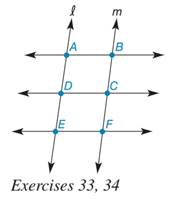Chapter 5.2, Problem 33EElementary Geometry For College St...

7th Edition
Alexander + 2 others
ISBN: 9781337614085

Solutions

Chapter
SectionElementary Geometry For College St...

7th Edition
Alexander + 2 others
ISBN: 9781337614085
Textbook Problem

In the drawing, A B ↔   | |     D C ↔     | |       E F ↔ with transversals l and m. If D and C are the midpoints of A E ¯ and B F ¯ , respectively, then is trapezoid ABCD similar to trapezoid DCFE?To determine

To check:

Whether the trapezoid ABCD is similar to DCFE or not

Explanation

Given:

In the figure, AB||DC||EF with transversals l and m. If D and C are the midpoints of AE¯ and BF¯.

Approach:

Two trapezoids are similar if and only if two conditions are satisfied:

1. Three or more parallel lines intercept congruent line segments on one transversal.

2. The intercept congruent line segments on any transversal.

Proof:

Let us consider the trapezoids, ABCD is similar to DCFE

Still sussing out bartleby?

Check out a sample textbook solution.

See a sample solution

The Solution to Your Study Problems

Bartleby provides explanations to thousands of textbook problems written by our experts, many with advanced degrees!

Get Started

Find f. f(x) = 5x4 3x2 + 4, f(1) = 2

Single Variable Calculus: Early Transcendentals, Volume I

(a) 715and121 (b) 38 and 57 (c) 2.6 and 1.8

Precalculus: Mathematics for Calculus (Standalone Book)

log27 9 =

Study Guide for Stewart's Single Variable Calculus: Early Transcendentals, 8th